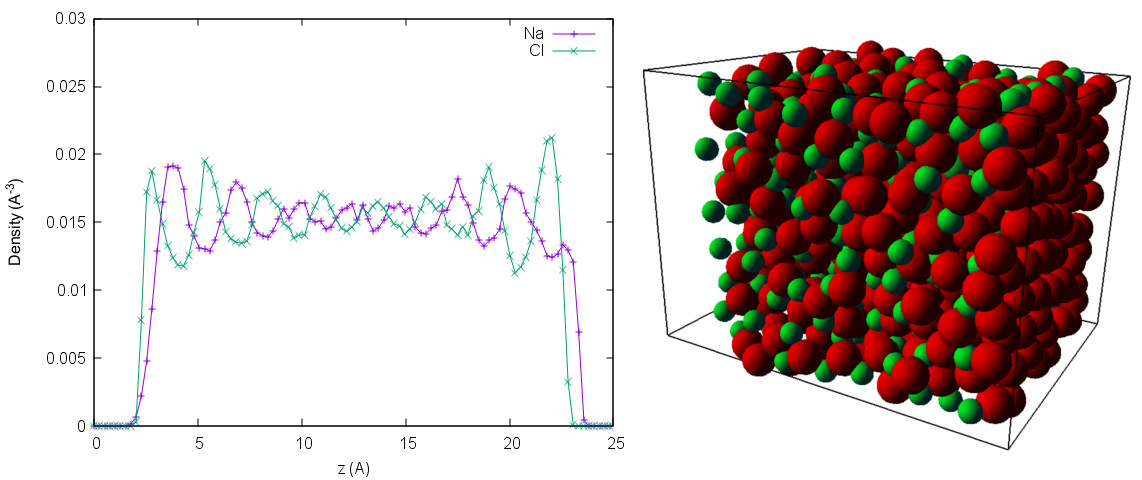# Tutorial 2: A Simple Charged System, Part 2¶

## 7 2D Electrostatics and Constraints¶

In this section, we use the parametrized NaCl system from the last task to simulate a molten salt in a parallel plate capacitor with and without applied electric field. We have to extend our simulation by several aspects:

### Confinement¶

ESPResSo features a number of basic shapes like cylinders, walls or spheres to simulate confined systems. Here, we use two walls at $z = 0$ and $z = L_z$ for the parallel plate setup ($L_z$: box length in $z$-direction)

### 2D-Electrostatics¶

ESPResSo also has a number of ways to account for the unwanted electrostatic interaction in the now non-periodic $z$-dimension. We use the 3D-periodic P$^3$M algorithm in combination with the Electrostatic Layer Correction (ELC). ELC subtracts the forces caused by the periodic images in the $z$-dimension. Another way would be to use the explicit 2D-electrostatics algorithm MMM2D, also available in ESPResSo.

### Electric Field¶

The simple geometry of the system allows us to treat an electric field in $z$-direction as a homogeneous force. Note that we use inert walls here and don't take into account the dielectric contrast caused by metal electrodes.

### Parameters¶

For our molten NaCl, we use a temperature $100 \ \mathrm{K}$ above the melting point ($1198.3 \ \mathrm{K}$) and an approximated density of $\rho = 1.1138 \ \mathrm{u \mathring{A}}$$^{-3}$ found in .

Let's walk through the python script. We need additional imports for the wall shapes and the ELC algorithm:

If we target a liquid system, we should not set up the particles in a lattice, as this introduces unwanted structure in the starting configuration. We define our system size by the number of particles and the density. The system parameters lead to the following values:

We save the force field parameters in python dictionaries, now with parameters for the walls:

To finally calculate the box size, we take into account the diameter of the electrode interaction. Additionally, ELC needs a particle-free gap in the $z$-direction behind the wall.

In the next snippet, we add the walls to our system. Our constraint takes two arguments: First the shape, in our case a simple plane defined by its normal vector and the distance from the origin, second the particle_type, which is used to set up the interaction between particles and constraints.

Now we place the particles at random position without overlap with the walls:

The scheme to set up the Lennard-Jones interaction is the same as before, extended by the Electrode-Ion interactions:

Next is the Lennard-Jones equilibration, followed by the thermostat:

As described, we use P$^3$M in combination with ELC to account for the 2D-periodicity. ELC is also added to the actors of the system and takes gap size and maximum pairwise errors as arguments.

For now, our electric field is zero, but we want to switch it on later. Here we run over all particles and set an external force on the charges caused by the field:

This is followed by our standard temperature equilibration:

In the integration loop, we like to measure the density profile for both ion species along the $z$-direction. We use a simple histogram analysis to accumulate the density data. Integration takes a while.

Finally, we calculate the average, normalize the data with the bin volume and save it to a file using NumPy's savetxt command.

Finally we can plot the density of the ions.

The resulting density plot is very noisy due to insufficient sampling, but should show a slight depletion of the smaller Na atoms at the walls. Now try to put in an electric field that represents an applied voltage of $15 \ \mathrm{V}$ between the walls and compare the results. The density data should show strong layering at the walls, decaying towards the system center. The complete script is at /doc/tutorials/02-charged_system/scripts/nacl_units_confined.py. In the interactive script nacl_units_confined_vis.py, you can increase/decrease the electric field with the keys u/j (at your own risk).Figure 4: Snapshot and densities along the $z$-axis with applied electric field for the ion species.

 Janz, G. J., Thermodynamic and Transport Properties of Molten Salts: Correlation Equations for Critically Evaluated Density, Surface Tension, Electrical Conductance, and Viscosity Data, J. Phys. Chem. Ref. Data, 17, Suppl. 2, 1988